# Rentals close to the slopes - Page 17

Viewing mode
350 results
• Slopes access :
• ski-in (-50 m)
• Rooms :
• 2 rooms
• 2 rooms + cabin(s)
• Total surface (sq.m) :
• 33 sq.m
•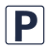•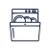•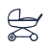•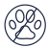• Slopes access :
• ski-in (-50 m)
• Rooms :
• Studio
• Total surface (sq.m) :
• 35 sq.m
•••• Slopes access :
• ski-in (-50 m)
• Rooms :
• Studio
• Total surface (sq.m) :
• 14 sq.m
•••• Slopes access :
• ski-in (-50 m)
• Rooms :
• 3 rooms
• 3 rooms + cabin(s)
• Total surface (sq.m) :
• 71 sq.m
•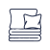•••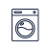••• Slopes access :
• ski-in (-50 m)
• Rooms :
• Studio + cabin(s)
• Total surface (sq.m) :
• 25 sq.m
••• Slopes access :
• ski-in (-50 m)
• Rooms :
• 2 rooms
• Total surface (sq.m) :
• 37 sq.m
••••• Slopes access :
• ski-in (-50 m)
• Rooms :
• Studio + cabin(s)
• Total surface (sq.m) :
• 30 sq.m
••••• Slopes access :
• ski-in (-50 m)
• Rooms :
• Studio
• Studio + cabin(s)
• Total surface (sq.m) :
• 30 sq.m
••• Slopes access :
• ski-in (-50 m)
• Rooms :
• Studio + cabin(s)
• Total surface (sq.m) :
• 32 sq.m
••••• Slopes access :
• ski-in (-50 m)
• Rooms :
• 4 rooms
• Total surface (sq.m) :
• 62-69 sq.m
•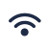•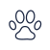••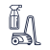••••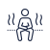•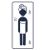• Slopes access :
• ski-in (-50 m)
• Rooms :
• Studio + cabin(s)
• Total surface (sq.m) :
• 29 sq.m
••••• Slopes access :
• ski-in (-50 m)
• Rooms :
• 2 rooms + cabin(s)
• Total surface (sq.m) :
• 40 sq.m
••••• Slopes access :
• ski-in (-50 m)
• Rooms :
• Studio + cabin(s)
• Total surface (sq.m) :
• 25 sq.m
•••• Slopes access :
• ski-in (-50 m)
• Rooms :
• Studio
• Total surface (sq.m) :
• 23 sq.m
••• Slopes access :
• ski-in (-50 m)
• Rooms :
• Studio
• Total surface (sq.m) :
• 30 sq.m
••••• Slopes access :
• ski-in (-50 m)
• Rooms :
• Studio + cabin(s)
• Total surface (sq.m) :
• 25 sq.m
••••• Slopes access :
• ski-in (-50 m)
• Rooms :
• Studio
• Studio + cabin(s)
• Total surface (sq.m) :
• 30 sq.m
••••• Slopes access :
• ski-in (-50 m)
• Rooms :
• Studio
• Studio + cabin(s)
• Total surface (sq.m) :
• 31 sq.m
••••• Slopes access :
• ski-in (-50 m)
• Rooms :
• Studio
• Studio + cabin(s)
• Total surface (sq.m) :
• 30 sq.m
•••••• Slopes access :
• ski-in (-50 m)
• Total surface (sq.m) :
• 55 sq.m
••••••